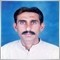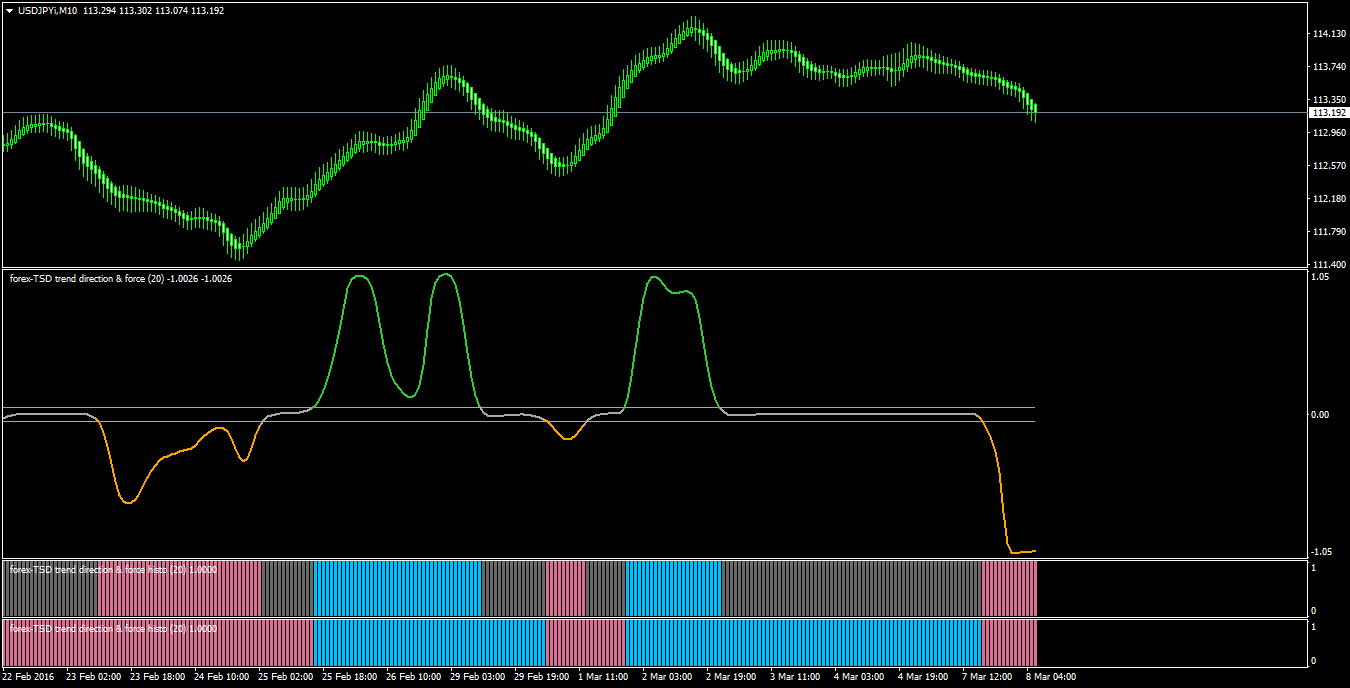Elite indicators :) - page 1174531

Wasn't there a "4 time frame " thread? I'm looking for a 4 time frame section or a 4 time frame RSI. The search function needs some attention.4534

Wasn't there a "4 time frame " thread? I'm looking for a 4 time frame section or a 4 time frame RSI. The search function needs some attention.

here are 2 versions of rsi 4 tf.

regards

4_time_frame_rsi_filter.mq4

4_time_frame_rsi_slope.mq4

Files:9496

Wasn't there a "4 time frame " thread? I'm looking for a 4 time frame section or a 4 time frame RSI. The search function needs some attention.

Ray, you can find the 4 tf thread here: https://www.mql5.com/en/forum/181187531

thanks fellows!72

I found this new indicator. Can someone convert it to work on mt4 please?

Files:1502

jenn9:
I found this new indicator. Can someone convert it to work on mt4 please?

That has coding errors4534

morro:
That has coding errors

and what about mq5 for mt4.:).151264

morro:
That has coding errors

Which errors?9496

Think maybe here

h=MA1;

l=MA1;

if (h<MA2) h=MA2;

if (h<MA3) h=MA3;

if (h<MA4) h=MA4;

if (h<MA5) h=MA5;

if (h<MA6) h=MA6;

if (h>MA2) h=MA2;

if (h>MA3) h=MA3;

if (h>MA4) h=MA4;

if (h>MA5) h=MA5;

if (h>MA6) h=MA6;

with h = high and l = low, thinking maybe the first part should maybe be

if (l<MA2) l=MA2;

if (l<MA3) l=MA3;

if (l<MA4) l=MA4;

if (l<MA5) l=MA5;

if (l<MA6) l=MA6;

but not sure!!

ps) from what I can tell the mq5 version came from this mt4 version from here:https://www.mql5.com/ru/code/7188187

mrtools:

NK Trade made the histo version, for the histo there is a trend mode, if trend mode = false you get the first histo, if true you get the bottom histo.

trend_direction__force_index_-_smoothed_4.30_histo__lines.ex4Dear mrtools ,

is the TREND MODE ON=TRUEon the histo version mean the same of the CHANGE THE COLOR ON ZERO LINE CROSS=TRUE on regular version ????